## Key Stage 2 results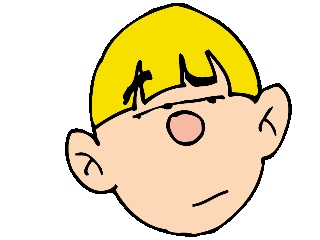Key Stage 2 test results which will be released next week are expected to show that almost 40% of 11 year olds ‘failed’ to hit the national targets in reading, writing and maths. What can we read into results such as these? Actually, very little!
Firstly, teachers at over 4000 schools (about a quarter) boycotted the tests and the NUT claim that this protest makes the results ‘an irrelevance’.
The expected standard at 11 is Level 4, but this has always been an unrealistic target for many children for many reasons. Why the government thinks that national testing improves education standards I do not know. From my own experience of 40 years teaching, real levels of achievement have not changed a great deal as a result of testing. They do tend to improve as a result of inspired teaching.
Perhaps what is more important is how little the Government makes of the data they collect. When making statements about how to improve education they would rather push for the latest fad ideas than for the result of any real evidence based research. This week the Centre for Policy Studies concludes that poor achievement in reading is a result of poor discipline and the absence of ‘synthetic phonics’ as a spelling programme. Yet they have done hardly anything to test this. The trials carried out have been tiny with under 200 children taking part – yet they collect all this data from Key Stage 2 test results from every school in the country, every year and fail to relate it to teaching methods to see what really does work.

## Coming soon: Addition, counting and calculator game for 7x tablesWhilst the Primary Framework for Mathematics has little to say about addition of whole numbers in Year 6 it is still worth revising skills and developing fast technique, especially with mental arithmetic.

Next week we have a consolidation page of adding three 2-digit numbers, using all the techniques developed so far. This is a follow up page to that published last week.

Whilst children get used to counting on in tens and hundreds, sometimes it can be useful to count on in 25s and 50s, especially when using money or measurement. We will be publishing the second page on counting in 25s and 50s, concentrating on money and is probably most suited to year 4 children.

There will also be something for Year 5. This is the next in our series of calculator activities and is certainly one of the hardest. It looks at multiples of 7, including numbers above 10 and will test children’s knowledge of the 7 times table to the full.

## Maths worksheet: More multiplying decimals by 10 and 100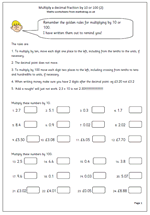Here we have another page on multiplying decimal fractions by 10 or 100. The method used today is to move each number one place to the left when multiplying by ten and two places to the left when multiplying by 100. The decimal point does not move. I seem to remember moving decimal points around when I was at school but this is certainly not recommended here.

When doing this with money it is worth remembering that two digits should always be written after the decimal point, so that £2.56 x 10 is not £25.6, but £25.60. (This is not an excuse for saying ‘add a nought’.)

Multiply a decimal by 10 and 100 (2)

## Year 3 worksheet: counting in tens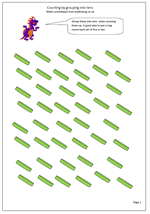These are a useful couple of pages for children entering Year 3 next term. Counting on and back will still be important in year 3, using up to three digit numbers. Grouping into tens or fives and using tally charts are both effective ways of counting larger numbers.

Children seldom get a chance to count a larger number of objects – somewhere between 30 and 60. By seeing and counting this many they become more able to estimate larger numbers and be able to give rough approximations rather than wild guesses.

Try similar exercises with heaps of coins, counters etc, dividing them up into fives or tens. A really good idea is to collect change (pennies etc) and guess how much of any one coin there is.

Counting by grouping into tens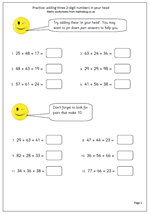Here we have a number of simple tips and practice at adding three 2-digit numbers mentally.

By Year 6 children should have a wide range of techniques at their fingertips for adding mentally.

starting with the largest digit

adding the tens before the units

looking for pairs that make 10

How to add three 2-digit numbers

## Coming soon: addition, counting and decimalsNext week we have a number of simple tips and practice at adding three 2-digit numbers mentally. Not the easiest task in the world and one beyond many adults, but there are a wide range of techniques children can have at their fingertips to make this easier.

starting with the largest digit

adding the tens before the units

looking for pairs that make 10

I will also be publishing a useful couple of pages for children entering Year 3 next term. Counting on and back will still be important in year 3, using up to three digit numbers. Grouping into tens or fives and using tally charts are both effective ways of counting larger numbers.

Also another page on multiplying decimal fractions by 10 or 100. The method used today is to move each number one place to the left when multiplying by ten and two places to the left when multiplying by 100. The decimal point does not move.

## Calculator game: 7x table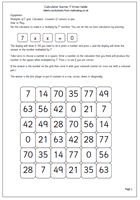Of all the tables, the 7x table is probably the least well known. The latest in our series of calculator games for two players helps with reinforcing the 7x table. Remember, as before, the aim of the game is to get four in a row, in any direction, before your opponent. A good knowledge of the 7x table will help with this.

This sequence of calculator games can be found in Year 3 and Year 4 Knowing Number Facts.

7x table calculator game

## Maths puzzle: One to nine (1)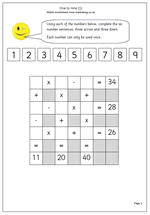Here is a tricky little puzzle which is aimed at Year 6 or upper juniors (9/11). Ideal for wet breaks!

Using just the digits 1 to 9 complete the number sentences, both across and down, so that they are all correct.

A good knowledge of tables is needed, together with the ability to add and subtract mentally.

The hardest part of this puzzle is getting started and the best approach is to use trial and improvement by picking one row or column which can only have a limited number of possibilities. This might well not be the first row across or column down.

Looking at this particular puzzle the first row is a x b – c = 34. Start by looking at combinations of numbers that when multiplied make more than 34 (but not more than 43 as the maximum to subtract is 9) and then see which number can be subtracted to leave 34.

## Year 6 maths worksheet: Decimal fractions (2)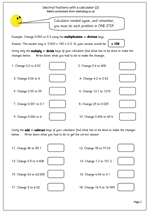A similar page to one posted earlier, this again looks at decimal fractions and how to manipulate them. The first set of questions involve changing decimals, using either the multiplication or division keys of the calculator, in just one step.

The second set of questions use the add or subtract keys.

Whilst the calculator is used, nevertheless a good understanding of number and place value is needed to be able to do these quickly and efficiently.

Decimals with a calculator (2)

## Resource of the Week: Year 5 division problems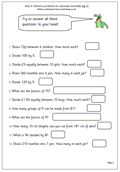As we get to the end of term here is a page which can be used to check that children can use a range of methods to solve division problems mentally.

It is important the tables are known so that the facts can be used. For example if it is known that 6 x 6 = 36, then 6 x 60 can be quickly worked out as 360, and in turn 360 divided by 6 is 60.

Another useful tip is when dividing by 5 mentally it is often possible to divide by 10 and then double the answer.